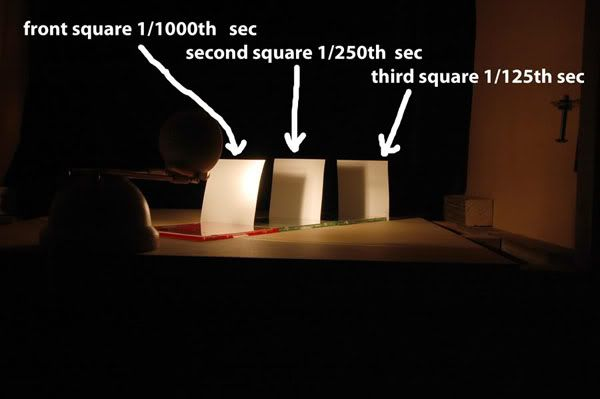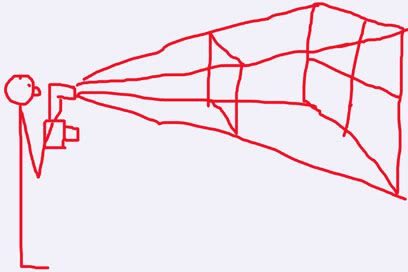## Wednesday, January 13, 2010

### Inverse square law

I know this isn't a perfect test but I decided to include some sort of illustration for the inverse square law and flash falloff .
We have three squares of paper , each 'one cd cover' away from the other and from the light source . The first one exposed at 1/1000th sec using spot metering . The second one at 1/250th [ 1/4 the brightness though it is only twice the distance from the light] .
Technically, with an even light source , the third one should have been 1/111th of a second but was 1/125th .The inverse square law states that twice the distance from the light = 1/2 squared = 1/4 the power [ needing 4X the exposure ] .
Three times the distance = 1/3 squared = 1/9 power [ needing 9X the exposure ] .
So the first one needed 1/1000th sec exposure , the second one needed 1/250th [ 4X as long ] and the third should have needed 1/111th sec [9X as long ] .

If a square is moved twice the distance away the sides look half their length , 1/2 the length X half the breadth = 1/4 the area .Three times the distance away they look 1/3 of their length and 1/3 X 1/3 = 1/9th the area .

So when we have twice the flash power we can work at 1.414X the distance [ 1.414squared = 2 ] . We would need 4X the flash power to work at twice the distance then
.